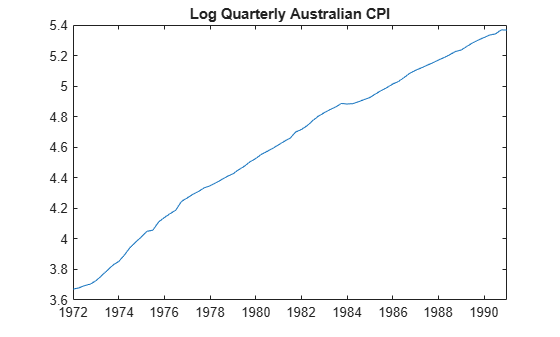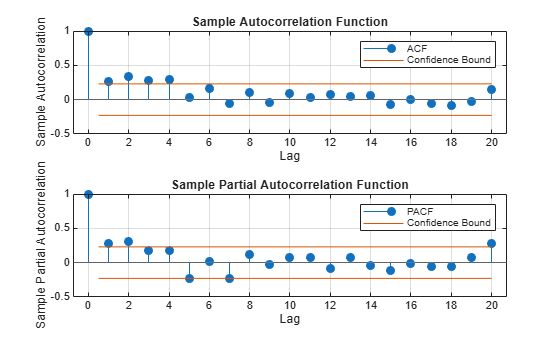Main Content

# Box-Jenkins Model Selection

This example shows how to use the Box-Jenkins methodology to select an ARIMA model. The time series is the log quarterly Australian Consumer Price Index (CPI) measured from 1972 and 1991.

### Load the Data

Load and plot the Australian CPI data.

```load Data_JAustralian y = DataTable.PAU; T = length(y); figure plot(y) h1 = gca; h1.XLim = [0,T]; h1.XTick = 1:10:T; h1.XTickLabel = datestr(dates(1:10:T),17); title('Log Quarterly Australian CPI')```The series is nonstationary, with a clear upward trend.

### Plot the Sample ACF and PACF

Plot the sample autocorrelation function (ACF) and partial autocorrelation function (PACF) for the CPI series.

```figure subplot(2,1,1) autocorr(y) subplot(2,1,2) parcorr(y)```The significant, linearly decaying sample ACF indicates a nonstationary process.

### Difference the Data

Take a first difference of the data, and plot the differenced series.

```dY = diff(y); figure plot(dY) h2 = gca; h2.XLim = [0,T]; h2.XTick = 1:10:T; h2.XTickLabel = datestr(dates(2:10:T),17); title('Differenced Log Quarterly Australian CPI')```Differencing removes the linear trend. The differenced series appears more stationary.

### Plot the Sample ACF and PACF of the Differenced Series

Plot the sample ACF and PACF of the differenced series to look for behavior more consistent with a stationary process.

```figure subplot(2,1,1) autocorr(dY) subplot(2,1,2) parcorr(dY)```The sample ACF of the differenced series decays more quickly. The sample PACF cuts off after lag 2. This behavior is consistent with a second-degree autoregressive (AR(2)) model.

### Specify and Estimate an ARIMA(2,1,0) Model

Specify, and then estimate, an ARIMA(2,1,0) model for the log quarterly Australian CPI. This model has one degree of nonseasonal differencing and two AR lags. By default, the innovation distribution is Gaussian with a constant variance.

```Mdl = arima(2,1,0); EstMdl = estimate(Mdl,y);```
``` ARIMA(2,1,0) Model (Gaussian Distribution): Value StandardError TStatistic PValue __________ _____________ __________ __________ Constant 0.010072 0.0032802 3.0707 0.0021356 AR{1} 0.21206 0.095428 2.2222 0.02627 AR{2} 0.33728 0.10378 3.2499 0.0011543 Variance 9.2302e-05 1.1112e-05 8.3066 9.8491e-17 ```

Both AR coefficients are significant at the 0.05 significance level.

### Check Goodness of Fit

Infer the residuals from the fitted model. Check that the residuals are normally distributed and uncorrelated.

```res = infer(EstMdl,y); figure subplot(2,2,1) plot(res./sqrt(EstMdl.Variance)) title('Standardized Residuals') subplot(2,2,2) qqplot(res) subplot(2,2,3) autocorr(res) subplot(2,2,4) parcorr(res) hvec = findall(gcf,'Type','axes'); set(hvec,'TitleFontSizeMultiplier',0.8,... 'LabelFontSizeMultiplier',0.8);```The residuals are reasonably normally distributed and uncorrelated.

### Generate Forecasts

Generate forecasts and approximate 95% forecast intervals for the next 4 years (16 quarters).

```[yF,yMSE] = forecast(EstMdl,16,y); UB = yF + 1.96*sqrt(yMSE); LB = yF - 1.96*sqrt(yMSE); figure h4 = plot(y,'Color',[.75,.75,.75]); hold on h5 = plot(78:93,yF,'r','LineWidth',2); h6 = plot(78:93,UB,'k--','LineWidth',1.5); plot(78:93,LB,'k--','LineWidth',1.5); fDates = [dates; dates(T) + cumsum(diff(dates(T-16:T)))]; h7 = gca; h7.XTick = 1:10:(T+16); h7.XTickLabel = datestr(fDates(1:10:end),17); legend([h4,h5,h6],'Log CPI','Forecast',... 'Forecast Interval','Location','Northwest') title('Log Australian CPI Forecast') hold off```References:

Box, G. E. P., G. M. Jenkins, and G. C. Reinsel. Time Series Analysis: Forecasting and Control. 3rd ed. Englewood Cliffs, NJ: Prentice Hall, 1994.

Download ebook